# Test: Even And Odd Integers- 2

## 15 Questions MCQ Test Quantitative Reasoning for GMAT | Test: Even And Odd Integers- 2

Description
Attempt Test: Even And Odd Integers- 2 | 15 questions in 30 minutes | Mock test for GMAT preparation | Free important questions MCQ to study Quantitative Reasoning for GMAT for GMAT Exam | Download free PDF with solutions
QUESTION: 1

Solution:
QUESTION: 2

### Which of the following statements must be true? I. The product of first 100 prime numbers is even II. The sum of first 100 prime numbers is odd. III. The sum of first five non-negative even numbers is divisible by both 4 and 5

Solution:

Explanation:- (a) The product of first 100 prime numbers will always be an even number , because it will contain 2 as a factor , since 2 is also a prime number

(b) 24,133 is the sum of the first 100 primes. Hence it is an odd number, so the statement is true

(c)The number series 2, 4, 6, 8, 10. Therefore, 40 is the sum of first 5 non negative even numbers. Hence it is divisible by 4 and 5

QUESTION: 3

### If x, y, z and w are positive integers, is x odd? (1) 7x + 8y + 4z + 5w is odd (2) 3x + 2y + 8z + 2w is even

Solution:
QUESTION: 4

If x and y are positive integers and x is odd, is xy even?

(1) x3y = 6a3 + 23 where a is a positive integer
(2) x2+y = 3k + 7 where k is a positive integer

Solution:
QUESTION: 5

If n is an integer, then which of the following statements is/are FALSE?

I. n3 – n is always even.

II. 8n3 +12n2 +6n +1 is always even.

III. √ (4n2 – 4n +1) is always odd.

Solution:
QUESTION: 6

If x and y are integers, is 3x4 + 4y even?

(1)  x3 is even

(2)  y2x + 3 is even

Solution:

Steps 1 & 2: Understand Question and Draw Inferences

We need to find if 3x4 + 4y is even.

4y will always be even, irrespective of the value of y, because an even number (4 here) multiplied by a number (whether even or odd) always gives an even product

-->  For the sum of 3x4 and 4y to be even, 3x4 must be even. This is because, the only way sum of two numbers can be even is if they are both even or both odd. Here, we have already determined that 4y is even.

3x4 is the product of an odd number (3) with x4

This product can be even only if x4 is even

x4 will be even if x is even

Therefore, in order to determine if 3x4 + 4y is even, we only need to find if x is even.

If x is even, 3x4 + 4y is even.

If x is odd, 3x4 + 4y is odd.

Step 3: Analyze Statement 1

x3 is even

-->  x is even

Sufficient

Step 4: Analyze Statement 2

y2x + 3 is even

-->  y2x is odd                                                                                                                              ( Odd + Odd = Even)

Now, an odd number multiplied with itself any number of times will give an odd product (similar result holds true for even numbers as well)

So, if y raised to the power something (2x in this case) is odd

-->  y is odd

We do not get to know if x is even or not.

Not Sufficient.

Step 5: Analyze Both Statements Together (if needed)

We get a unique answer in step 3, so this step is not required

QUESTION: 7

If X is a positive integer, is X2 + 1 an odd number?

(1) X is the smallest integer that is divisible by all integers from 11 to 15, inclusive.

(2) 3X is an odd number.

Solution:

Steps 1 & 2: Understand Question and Draw Inferences

For X2 + 1 to be odd

--> X2 must be even

-->  X must be even

We need to find if X is even or not

Step 3: Analyze Statement 1

X is the smallest integer that is divisible by all integers from 11 to 15, inclusive

--> X is divisible by 12 and 14

-->  X is even

Sufficient.

Step 4: Analyze Statement 2

3X is an odd number

An odd number multiplied with itself any number of times will give an odd product

--> 3X will always be odd, whether X is even or odd

Not Sufficient.

Step 5: Analyze Both Statements Together (if needed)

We get a unique answer in step 3, so this step is not required

QUESTION: 8

If a, b, and k are positive integers, is the sum (a + b) an even number or an odd number?

(1) a = ( k3 + 3k2 + 3k + 6)

(2) b = (k2 + 4a +5)

Solution:

Steps 1 & 2: Understand Question and Draw Inferences

We are given that a, b, and k are positive integers. And, we have to find out whether the sum of a and b will be even or odd.

Let us draw a table for all the possible cases for the even-odd property of a and b, and the corresponding property of the sum (a+b):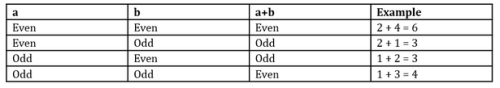Thus we see that:
If a and b are both odd or both even, then the sum (a+b) will be even

But if one out of a and b is even, and the other is odd, the sum (a+b) will be odd.

So, we will need to determine the even-odd property of both a and b in order to be in a position to say whether the sum (a+b) is odd or even.

Step 3: Analyze Statement 1

Let’s analyze statement I:

a = k3 + 3k2 + 3k + 6

As we can see the value of a depends on the value of k.  There can be two cases for the value of k – k can be either odd or even:

1. If k is odd:

This means, k3 = Odd    (Odd*Odd*Odd = Odd; Think: 3*3*3 = 27)

Similarly, k2 will also be odd

So, 3k2 = Odd    (Since 3 is odd and Odd*Odd = Odd)

Also, 3k = Odd

So, we get that

a = Odd number + odd number + odd number + even number

Think of easier numbers of the type of the right hand side of the above equation:

1 + 1 + 1 + 2 = 5, which is odd

This means that the RHS of the above equation will be an odd number

So, a is an odd number

So, if k is an odd number, a will be an odd number.

2. If k is even:

This means, k3 = Even   (Even*Even*Even = Even; Think: 2*2*2 = 8)

Similarly, k2 will also be even

So, 3k2 = Even   (Odd*Even = Even)

Also, 3k = Even

So, we get that

a = Even number + even number + even number + even number =  Even number

So, a is an even number

Thus, if k is an even number, “a” will be an even number.

To conclude,

If k is odd => a is odd

If k is even => a is even

Thus, the value of a can be even or odd, depending on the value of k. This step fails to give us a definite answer whether a is an even number or odd number. Also, it does not give us any information about the values of b.

So, we can’t determine whether the expression a + b will be even or odd.

So, Statement 1 alone is not sufficient to arrive at a unique answer.

Step 4: Analyze Statement 2

Moving on, let’s analyze statement II:

b = (k2 + 4a +5)    …………… (2)

Now, the value of b depends on the values of a and k.

If the values of a and k are independent of each other, then, we will have to consider 4 cases for the value of b: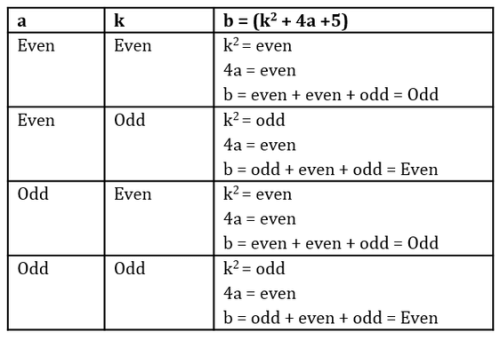Thus, we see that:

i)                When k is even, b is odd

ii)               When k is odd, b is even

iii)             The even-odd property of b doesn’t depend on the value of a

Now, it is possible that the values of k and a are dependent on one another.

For example, if you were given that k = 8a

This would mean that irrespective of whether a is even or odd, k will always be even.

In that case, you would have been able to determine for sure that b will always be odd (though you would still be clueless about whether a is odd or even).

Or, if you were given that a = 8k, you would have been able to determine that a is even no matter what the value of k.

We are not given any information about the relationship, if any, between k and a. Neither are we given any other clue about the even-odd property of a.

Since this statement doesn’t tell us whether:

i)                k is even or odd

ii)               a is even or odd

iii)             a and k are independent or not,

we will not be able to determine whether the sum (a+b) is even or odd.

So, Statement 2 alone is not sufficient to arrive at a unique answer

Step 5: Analyze Both Statements Together (if needed)

Since the individual analysis of the statements does not yield us any significant result, let’s analyse both of them together:

From Statement 1:

If k is odd => a is odd

If k is even => a is even

From Statement 2:

When k is even, b is odd

When k is odd, b is even

Combining the two statements, we get the following table: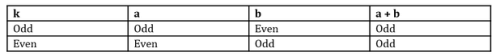Thus, we see that irrespective of the value of k, the sum (a+b) is always odd.

Thus, we have been able to arrive at a unique solution by combining the two statements.

QUESTION: 9

If x and y are integers, is y even?

(1)  (x + 2) * (y2 + 7) is even

(2)  (x3 + 8) * (y2 -4) is even

Solution:

Step 1 and 2: Understand the question and draw inferences

We are given that x and y are integers, and we are asked if y is even. Since we do not have any more information to draw inferences from, let’s move straight to Statement 1.

Step 3: Analyze Statement 1

(x + 2) * (y2 + 7) is even

The product of two numbers is even if at least one of the two numbers is even.

Thus, three possibilities arise: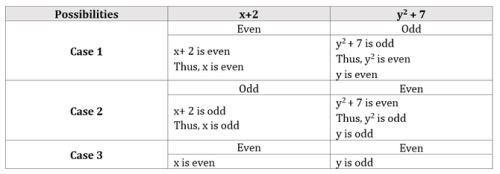Statement 1 holds true for each of these 3 cases. Thus, we cannot ascertain for sure if y is odd or even.

Therefore, Statement 1 alone is not sufficient to answer the question.

Step 4: Analyze Statement 2

(x3 + 8) * (y2 -4) is even

Again, the product of two numbers is even if at least one of the two numbers is even.

So, three possibilities arise for this statement as well: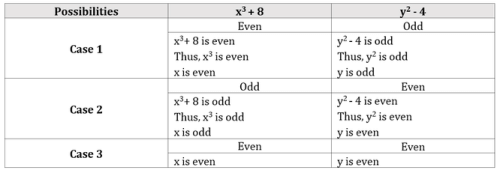Statement 2 holds true for each of these 3 cases. Thus, we cannot ascertain for sure if y is odd or even.

Therefore, Statement 2 alone is not sufficient to answer the question.

Step 5: Analyze both statements together (if needed)

We have seen that

Statement 1 holds true if:

i)   x is even, y is even or odd

ii)  x is odd, y is odd

Statement 2 holds true if:

i)   x is even, y is even or odd

ii)  x is odd, y is even

Both Statements will be true at the same time only if:

x is even, y is even or odd.

Thus, even after combining both the statements, we have not been able to ascertain the even-odd nature of y.

Thus, both the statements together are not sufficient to answer the question.

QUESTION: 10

If x, y, and z are positive integers, where x is an odd number and z = x2 + y2 + 4. Is y2 divisible by 4?

(1) Z = 8k -3  where k is a positive integer

(2) When (z-x+1) is divided by 2, it leaves a remainder.

Solution:

Steps 1 & 2: Understand Question and Draw Inferences

We are given that x, y and z are positive integers.

Also, x is odd

And,

z= x2 + y2 + 4

By rearranging the terms, we get:

y2 = z – x2 – 4            ………….  (1)

The question asks us whether y2 is divisible by 4 or not. If y is divisible by 2, then y2 will be divisible by 4; else it will not be.

And if y is divisible by 2, this means that y is even.

Thus, the question is indirectly asking us: is y even?

y will be even if the right hand side of Equation 1 is even. So, let’s analyze the condition in which this will be so.

Now, we are given that x is an odd number. This means that x2 is also odd (Odd * Odd = Odd)

So, in the part (x2 – 4), we are subtracting an even number from an odd number. Think of what happens when we subtract 4 from 9. We get 5, which is an odd number. So, here too, the result of subtracting 4 from x2 will be an Odd number (Please note that the result may be positive or negative. We are not concerned with that. All that we want to know is whether the result will be even or odd)

So, from Equation 1 we get:

y2  = z – Odd number . . . (2)

Now,

Even +/-Odd = Odd   (Think: 4 + 1 = 5)

And, Odd +/-Odd = Even   (Think: 3 + 1 = 4)

Thus, from equation (2)

It is clear that y2 will be even only if z is an odd number

• y will be even only if z is an odd number
• Our task is to determine if z is odd or not.

Step 3: Analyze Statement 1

Statement (1) says:

z = 8*k – 3

Now, irrespective of whether k is even or odd,

8 * k = even number

ð  8k – 3 = Even number -odd number = Odd Number   (Think: 8 – 3 = 5)

So, the analysis of statement (1) tells us that z is an odd number.

Since we have been able to determine that z is an odd number, this means that y is an even number.

Thus, Statement (1) alone is sufficient to answer the question: is y even?

Step 4: Analyze Statement 2

When the expression (z-x+1) is divided by 2, we get a remainder. This tells us that (z-x+1) is an odd number. So,

z – x + 1 = odd

This means that,

z – x = Odd number – 1 = Odd number – odd number = Even number   (Think: 5 – 3 = 2)

So, z = x + Even number

Now, we are given that x is an odd number

So, z = Odd number + Even number = Odd number   (Think: 1 + 2 = 3)

Thus, z is an odd number

• y is an even number

Thus, Statement 2 alone is sufficient to answer the question: is y even?

Step 5: Analyze Both Statements Together (if needed)

Since we got unique answers in step 3 and 4, so this step is not required

QUESTION: 11

If A is a positive integer, then which of the following statements is true?

1.  A2 + A -1 is always even.

2.  (A4+1)(A4+2) + 3A is even only when A is even.

3.  (A-1)(A+2)(A+4) is never odd.

Solution:

Step 1: Question statement and Inferences

We are given that A is a positive integer. A may be odd or even.

Step 2: Analyzing the given statements

1.       A2 + A -1 is always  even

Case (i) A is Odd

• A2 is also odd    (Odd × Odd = Odd)
• A2 + A is even   (Odd + Odd = Even)
• A2 + A -1 is odd   ( Even – Odd = Odd)

We don’t even need to consider the case when A is even, because Statement 1 has already been proven FALSE.

2.       (A4+1)(A4+2) + 3A is even only when A is even

Case (i): A is odd

• 3A is odd    (Odd × Odd = Odd)

Now, an odd number multiplied with itself any number of times will give an odd product

• A4 is odd
• A4 + 1 is even     (Odd + Odd = Even)
• A4 + 2 is odd       (Odd + Even = Odd)
• (A4+1)(A4+2) + 3A = Even × Odd + Odd
• (A4+1)(A4+2) + 3A = Even  + Odd
• (A4+1)(A4+2) + 3A = Odd

Thus, when A is odd, (A4+1)(A4+2) + 3A is odd

Case (ii): A is even

3A is even                (Odd × Even = Even)

An even number multiplied with itself any number of times will give an even product

• A4 is even
• A4 + 1 is odd    (Even + Odd = Odd)
• A4 + 2 is even    (Even + Even = Even)
• (A4+1)(A4+2) + 3A = Odd × Even + Even
• (A4+1)(A4+2) + 3A = Even  + Even
• (A4+1)(A4+2) + 3A = Even

Thus, when A is even, (A4+1)(A4+2) + 3A is even

Thus, Statement 2 is TRUE.

3.       (A-1)(A+2)(A+4) is never odd

Case (i): A is odd

•  A-1 is even                                       (Odd -Odd = Even)
• A + 2 is odd                                       (Odd + Even = Odd)
• A + 4 is odd                                       (Odd + Even = Odd)
• (A-1)(A+2)(A+4) = Even × Odd × Odd
• (A-1)(A+2)(A+4) = Even

Thus, when A is odd, (A-1)(A+2)(A+4) is even

Case (ii): A is even

• A-1 is odd                                          (Even -Odd = Odd)
• A + 2 is even                                     (Even + Even = Even)
• A + 4 is even                                     (Even + Even = Even)
• (A-1)(A+2)(A+4) = Odd × Even × Even
• (A-1)(A+2)(A+4) = Even

Thus, when A is even, (A-1)(A+2)(A+4) is even

Thus, Statement 3 is TRUE.

QUESTION: 12

If a, b, c, d, and e are integers and the expression (ab2c2 /d2e) gives a positive even integer, which of the following options must be true?

I.  abc is even

II.  a/e is positive

III.  a/d2 is positive

Solution:

Step 1: Question statement and Inferences

We are given that a, b, c, d, and e are integers.

Also, it is given that

ab2c2 /d2e = positive even number          . . .. (1)

We can also write this expression as:

ab2c2 = (Positive Even number)*(d2e)

When you multiply any number, whether it is even or odd, with an even number, the product is always even.

So, we can deduce that:

• ab2c2 = even

Also, equation (1) can be written as:

(b2c2 /d2) * (a/e) = positive even number

Now, we know that the product of two numbers can be positive only if both are positive or both are negative:                      (Think;    2*3 = 6   OR    -2 * -3 = 6)

And in the above expression, (b2c2 /d2) is always positive since even powers of an integer are always positive.

Since the first number (b2c2 /d2) is always positive, the second number (a/e) also needs to be positive.

(Note that, here it is possible that a and e both are negative, but the overall expression (a/e) is definitely positive)

So, we know that a/e is positive.

Step 2: Finding required values

Now, let’s move on to the analysis of the options:

I. abc is even.

As we know, the expression (ab2c2) is even and it can be written as:

ab2c2 = a*b*b*c*c

Now, since the above expression yields an even number, it tells us that at least one of the integers a, b, or c is an even number.   (Think 2 * 3 * 5 = 30)

Now, if at least one of the integers a, b, or c is an even number then the product (a*b*c) will also be an even number.

So, this statement is always true.

II. a/e is positive

We already proved that a/e is positive.

So, this statement is always true.     III. a/d2 is positive

In the expression a/d2 since d2 is a square form, it is always positive. However, the question doesn’t state whether a is positive or negative. So, we can’t clearly say whether a/d2 is positive or negative.

So, we can’t say that this statement is always true.

Step 3: Calculating the final answer

Hence, the options that are always true are I and II. So, the correct answer choice is choice C.

Take Away

1. When a fraction after simplification can be represented as an even integer, it implies that the numerator of that fraction is an even integer.
2. If a multiplication expression yields a positive product, it means that the number of negative numbers in the expression is even. It can be zero, two, four etc. but it will never be an odd number. For example:

(-2) * (-1) * (3) = 6

(-3) * (4) * (5) = -60

QUESTION: 13

If r and s are positive integers, and r2 + r/s is an odd integer, which of the following cannot be even?

Solution:

Step 1: Question statement and Inferences

Given:   r and s are positive integers

r2 + r/s is odd

To Infer: Let’s try to infer whether r and s are even or odd.

Let’s first consider the possibility that r is odd.

Case (i): r is odd

-->  r2 is odd                                                                                                              (Odd × Odd = Odd)

Now,      r2 + r/s = Odd

-->  Odd + r/s = Odd

-->  r/s   = Even                                                                                                          (Odd -Odd = Even)

-->  Odd/s = Even

-->  s × Even = Odd

Not possible! When one of the numbers being multiplied is even, the product is always even

Conclusion: r cannot be odd

-->  r must be even

Let’s also see if we can determine whether s is even or odd.

We know now that r is even

-->  r2 is even                                                                                                             (Odd × Odd = Odd)

Now,      r2 + r/s = Odd

-->  Even + r/s = Odd

-->  r/s   = Odd                                                                                                            (Odd -Even = Odd)

-->  Even/s = Odd

-->  s × Odd = Even

-->  s is Even

Conclusion: Both r and s are even integers

Step 2: Analyzing the given options

• 3r + 2s

Both r and s are even

-->  3r is even                                                                                                            (Odd × Even = Even)

2s is even                                                                                                                 (Even × Even = Even)

-->  3r + 2s is even                                                                                                    (Even + Even = Even)

• (r-1)(s+2)

Both r and s are even

-->  r-1 is odd                                                                                                             (Even -Odd = Odd)

s + 2 is even                                                                                                             (Even + Even = Even)

-->  (r-1)(s+2) is even                                                                                                 (Odd × Even = Even)

• rs+1 + sr

Both r and s are even

An even number multiplied with itself any number of times will give an even product

-->  rs+1  is even

sr  is even

-->  rs+1 + sr is even

• r3 +3

r is even

-->  r3 is even

-->  r3 + 3 is odd

We don’t need to analyze Option E since we have already found the expression that cannot be even.

Step 3: Calculating the final answer

Among the given options, Option D cannot be even

QUESTION: 14

Which one of the following is odd?

Solution:

Step 1:

8 × 4 = 32
6 × 7 = 42
9 × 6 = 54

Step 2:
Only 11 × 3 = 33 is odd. Rest are even.

QUESTION: 15

Which one of the following is even?

Solution:

Step 1:

3 x 9 = 27
5 x 7 = 35
5 x 3 = 15

Step 2:
Only 9 × 8 = 72 is even. Rest are odd.Use Code STAYHOME200 and get INR 200 additional OFF Use Coupon Code